Question Pool Similar Triangles

# Similar Triangles - SAMAGRA Question Pool & Answers | Class 9 English Medium

Kerala Syllabus SAMAGRA SCERT SAMAGRA Question Pool for Class 9 English Medium Maths Similar TrianglesQn 1.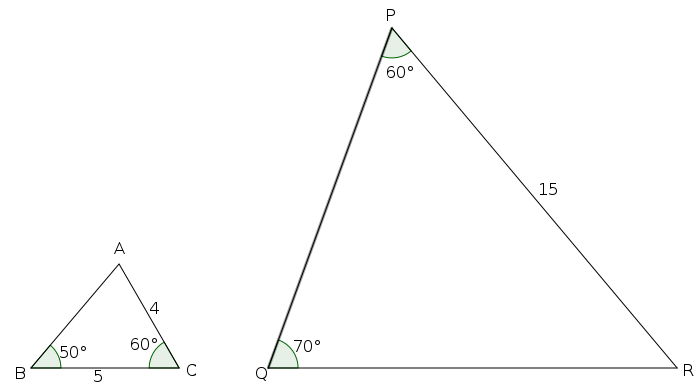a) Write the measure of ∠A.

b) Find the length of PQ?

∠A

Get Free Study Materials + 1 Week Free Trial of BrainsPrep Class 9 English Medium Tuition

Qn 2.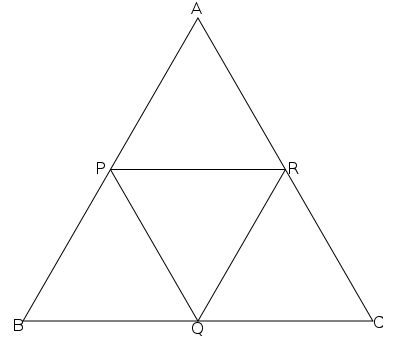In the figure, triangle ABC is an equilateral triangle. P,Q,R are the mid points of the sides AB,BC, CA respectively.

a) Find the measures of the angles of triangle PBQ

b) Find the measures of the angles of triangle PQR

c) Prove that the lengths of the sides of triangle PQR are half the lengths of sides of triangle ABC.

a) 60° each

b) 60°

Get Free Study Materials + 1 Week Free Trial of BrainsPrep Class 9 English Medium Tuition

Qn 3.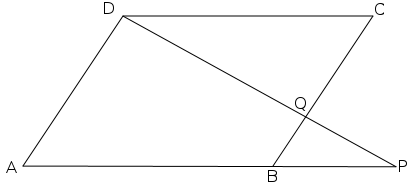In the figure ABCD is a parallelogram. QC=2BQ.

a) Prove that triangles PQB and CQD are similar.

b) How many times the sides of triangle PBQ are the sides of triangle CQD.

c) Find another pair of similar triangles from the figure.

a) ∠ CQD =∠ PQB

∠ CDQ = ∠QPB

∠ DCQ = ∠PBQ . triangles are similar.

Get Free Study Materials + 1 Week Free Trial of BrainsPrep Class 9 English Medium Tuition

Qn 4.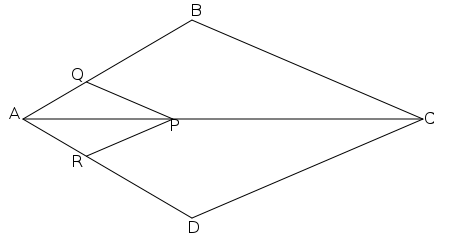In the figure PQ is parallel to BC and PR is parallel to CD

a) Write the equal angles of triangles AQP and ABC

b) Write the equal angles of triangles ARP and ADC

c) Prove that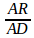=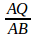Get Free Study Materials + 1 Week Free Trial of BrainsPrep Class 9 English Medium Tuition

Qn 5.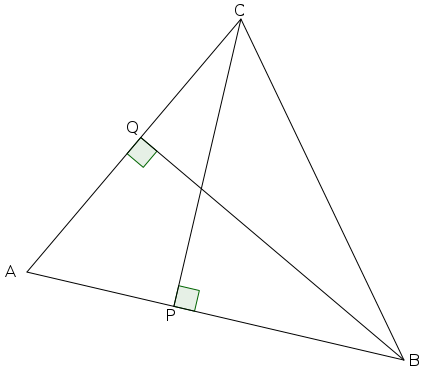In triangle ABC, CP and BQ are perpendicular to the sides AB and AC respectively.

a) If ∠A=x°, find the measures of ∠ABQ and ∠ACP.

b) Write the ratios of the sides opposite to the equal angles in triangle AQB and triangle APC

c) Prove that AP x AB = AQxAC

Get Free Study Materials + 1 Week Free Trial of BrainsPrep Class 9 English Medium Tuition

Qn 6.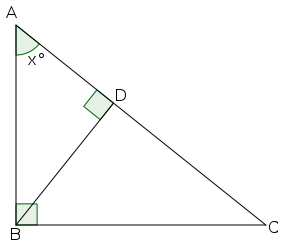In triangle ABC, ∠B=90°. BD is perpendicular to AC.

a) If ∠A=x° , find the measures of ∠C and ∠ABD .

b) Write the ratios of the sides opposite to the equal angles in triangle ABD and triangle ABC

c) Prove that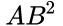= AD x AC